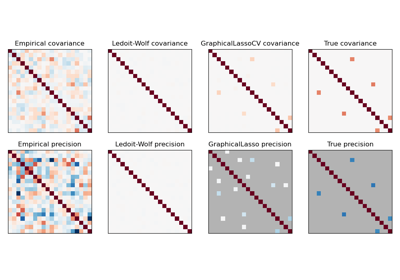# sklearn.datasets.make_sparse_spd_matrix¶

sklearn.datasets.make_sparse_spd_matrix(dim=1, *, alpha=0.95, norm_diag=False, smallest_coef=0.1, largest_coef=0.9, random_state=None)[source]

Generate a sparse symmetric definite positive matrix.

Read more in the User Guide.

Parameters:
dimint, default=1

The size of the random matrix to generate.

alphafloat, default=0.95

The probability that a coefficient is zero (see notes). Larger values enforce more sparsity. The value should be in the range 0 and 1.

norm_diagbool, default=False

Whether to normalize the output matrix to make the leading diagonal elements all 1.

smallest_coeffloat, default=0.1

The value of the smallest coefficient between 0 and 1.

largest_coeffloat, default=0.9

The value of the largest coefficient between 0 and 1.

random_stateint, RandomState instance or None, default=None

Determines random number generation for dataset creation. Pass an int for reproducible output across multiple function calls. See Glossary.

Returns:
precsparse matrix of shape (dim, dim)

The generated matrix.

make_spd_matrix

Generate a random symmetric, positive-definite matrix.

Notes

The sparsity is actually imposed on the cholesky factor of the matrix. Thus alpha does not translate directly into the filling fraction of the matrix itself.

## Examples using sklearn.datasets.make_sparse_spd_matrix¶Sparse inverse covariance estimation

Sparse inverse covariance estimation